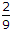# Civil Engineering - UPSC Civil Service Exam Questions

41.

What is the total degree of indeterminancy, both internal and external of the plane frame ?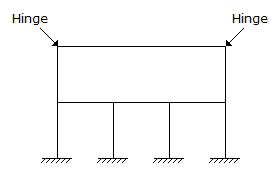A. 10 B. 11 C. 12 D. 14

Explanation:

No answer description available for this question. Let us discuss.

42.

In the design of two-way slab restrained at all edges, torsional reinforcement required is :

 A. 0.75 times the area of steel provided at midspan in the same direction B. 0.375 times the area of steel provided at midspan in the same direction C. 0.375 times the area of steel provided in the shorter span D. Nil

Explanation:

No answer description available for this question. Let us discuss.

43.

Two bars AO and BO are of uniform area 'A' each and are hinged at O as shown in the given figure. A load W is applied at O. The vertical deflection of O will be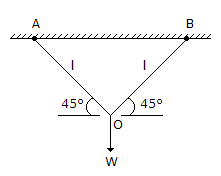A.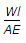B.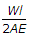C.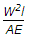D.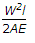Explanation:

No answer description available for this question. Let us discuss.

44.

The length of a transition curve for a circular curve of radius 300 m and for a design speed of 15 m/s, when the rate of change of centrifugal acceleration is 0.3 m/s3 is

 A. 30 m B. 37.5 m C. 45 m D. 60 m

Explanation:

No answer description available for this question. Let us discuss.

45.

Two closed-coil springs of stiffness s and 2s are arranged in series in one case and in parallel in the other case. The ratio of stiffness of springs connected in series to parallel is

 A.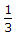B.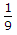C.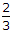D.